Click to Chat

1800-1023-196

+91-120-4616500

CART 0

• 0

MY CART (5)

Use Coupon: CART20 and get 20% off on all online Study Material

ITEM
DETAILS
MRP
DISCOUNT
FINAL PRICE
Total Price: Rs.

There are no items in this cart.
Continue Shopping```Matrices: Definition and Classification

Table of Content

Definition of Materices and Classification

Classification of Matarices

Traces of a Matrix

Diagonal Matrix

Properties of Transpose

Properties of Conjugate

​Transpose Conjugate of a Matrix

Definition of Materices and ClassificationA rectangular array of symbols (which could be real or complex numbers) along rows and columns is called a matrix.

Thus a system m × n symbols arranged in a rectangular formation along m rows and n columns and bounded by the brackets [.] is called an m by n matrix (which is written as m x n matrix)

i.e. A =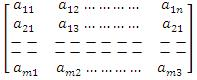is a matrix of order m × n.

In a compact form the above matrix is represented by A = [aij], 1 < i < m,
1 < j < n, where is, j ε i or simply [aij] m × n.

The numbers a11, a12, ... etc of this rectangular array are called the elements of the matrix. The element aij belongs to the ith row and the jth column and is called the (i, j)th element of the matrix.

Equal Matrices

Two matrices are said to be equal if they have the same order and each element of one is equal to the corresponding element of the other.

Classification of Matarices

Row Matrix

A matrix having a single row is called a row matrix. e.g. [1 3, 5, 7]

Column Matrix

A matrix having a single column is called a column matrix. e.g.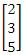.

Square Matrix

An m x n matrix A is said to be a square matrix if m = n i.e. number of rows = number of columns.

For Example: A =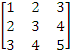is a square matrix of order 3 × 3.

Note:  In a square matrix the diagonal from left hand side upper corner to right hand side lower corner is known as leading diagonal or principal diagonal. In the above example square matrix containing the elements 1, 3, 5 is called the leading or principal diagonal.

Traces of a Matrix

The sum of the elements of a square matrix A lying along the principal diagonal is called the trace of A i.e. tr(A). Thus if A = [aij]n×n,

then tr(A) = ∑ni=1  aii = a11 + a22 +......+ ann.

Diagonal Matrix

A square matrix all of whose elements except those in the leading diagonal, are zero is called a diagonal matrix. For a square matrix A = [aij]n×n to be a diagonal matrix, aij = 0, whenever i ≠ j.

For example: A =is a diagonal matrix of order 3 × 3.

Note:  Here A can also be represented as diag (3,5 -1).

Scalar Matrix

A diagonal matrix whose all the elements are equal is called a scalar matrix.

For a square matrix A = [aij]n×n to be a scalar matrix, aij =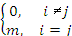, where m ≠ 0.

For example: A =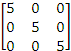is a scalar matrix.

Unit Matrix or Identity Matrix

A diagonal matrix of order n which has unity for all its elements, is called a unit matrix of order n and is denoted by In.

Thus a square matrix A = [aij]n×n is a unit matrix if aij =.

For example :

I3 =.

Triangular Matrix

A square matrix in which all the elements below the principal diagonal are zero is called Upper Triangular matrix and a square matrix in which all the elements above the principal diagonal are zero is called Lower Triangular matrix.

Given a square matrix A = [aij]n×n,

for upper triangular matrix, aij = 0,       i > j

and for lower triangular matrix, aij = 0,   i < j.

Note :

Diagonal matrix is both upper and lower triangular

A triangular matrix A = [aij]n×n is called strictly triangular if aii = 0 for 1 < i < n.

For Example :are respectively upper and lower triangular matrices.

Null Matrix

If all the elements of a matrix (square or rectangular) are zero, it is called a null or zero matrix.

For A = [aij] to be null matrix, aij = 0i, j.

For Example: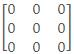is a zero matrix.

Transpose of a Matrix

The matrix obtained from any given matrix A, by interchanging its rows and columns, is called the transpose of A and is denoted by A'.

If A = [aij]m×n and A' = [bij]n×m then bij = aij,i, j.

For Example:

If A =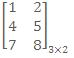, then A' =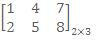Properties of Transpose

(i)     (A')' = A

(ii)    (A + B)' = A' + B', A and B being conformable matrices

(iii)   (αA)' = αA', α being scalar

(iv)   (AB)' = B'A', A and B being conformable for multiplication

Conjugate of a Matrix

The matrix obtained from any given matrix A containing complex number as its elements, on replacing its elements by the corresponding conjugate complex numbers is called conjugate of A and is denoted by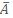.

For Example:

A =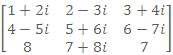. Then,Properties of Conjugate​Transpose Conjugate of a Matrix

The transpose of the conjugate of a matrix is called transposed conjugate of A and is denoted by Aq. The conjugate of the transpose of A is the same as the transpose of the conjugate of A i.e.= AΘ.

If A = [aij]m×n, then AΘ = [bij]n×m where bji =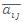i.e. the (j, i)th element of AΘ = the conjugate of (i, j)th element of A.

For Example:

If A =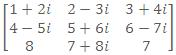then AΘ =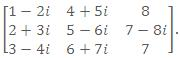.

Properties of Transpose Conjugate

(i)    (AΘ)Θ = A

(ii)   (A + B)Θ = AΘ + BΘ

(iii)   (kA)Θ =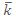AΘ, k being any number

(iv)   (AB)Θ = BΘAΘ
The platform at askIITians is the only group of IITians which provide you free online courses and a good professional advice for IIT JEE, AIEEE and other Engineering Examination preparation. You can also visit askIITians.com to study topics pertaining to the IIT JEE and AIEEE syllabus for free.

To read more, Buy study materials of Matrices and Determinants comprising study notes, revision notes, video lectures, previous year solved questions etc. Also browse for more study materials on Mathematics here.
```### Course Features

• 731 Video Lectures
• Revision Notes
• Previous Year Papers
• Mind Map
• Study Planner
• NCERT Solutions
• Discussion Forum
• Test paper with Video Solution